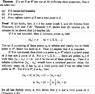# Heine-borel related proof

bedi
I'm trying to understand that proof with little success. Particularly I don't understand why "S has x_0 as a limit point, and S has no other limit point in R^k". Please help

#### Attachments

•photo (1).JPG
70.5 KB · Views: 606

Staff Emeritus
Homework Helper
The conditions $|x_n-x_0|<1/n$ imply that $(x_n)_n$ converges to $x_0$. Thus $x_0$ is a limit point of the $(x_n)_n$ (which is the set S). Since limits of sequences are unique in $\mathbb{R}^k$, the limit point $x_0$ is unique.

bedi
Alright, but can you explain it with basic topology, like neighbourhoods and the definition of a limit point? I don't see why any other interior point of S couldn't be a also a limit point in that case. Rudin says that it's because it would violate the triangular inequality theorem, but still it's not clear enough...

Staff Emeritus
Homework Helper
If y is a limit point of $(x_n)_n$, then there exists a subsequence $(x_{k_n})_n$ that converges to y. But since the original sequence $(x_n)_n$ converges to x, the subsequence must also converge to x.

So $(x_{k_n})_n$ converges to both x and y. Thus x must equal y.